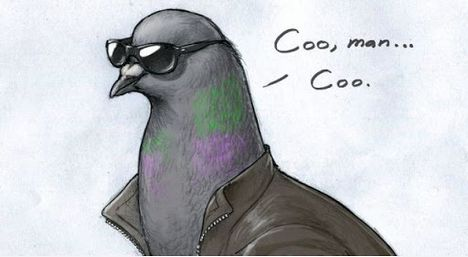# Binary PigeonssakibalaminNSUPS Bootcamp Season 07...
Limits 1s, 512 MB

There are $N$ pigeons standing one after another in a straight line. There are two kinds of pigeons: white pigeon ($\texttt{W}$) and black pigeon ($\texttt{B}$). We can represent the ordering of pigeons by string ($S$). For example, $\texttt{WWB}$ means a white pigeon is standing in the first position, another white pigeon is standing in the second position and a black pigeon is standing in the third position.Now you are given some extra pigeons, let's say $\texttt{B}$ black pigeons and $\texttt{W}$ white pigeons. You have to place the extra pigeons in the original string such that the new order will not have any adjacent pigeons with the same color. If it's possible then print $\texttt{YES}$, otherwise print $\texttt{NO}$.

## Input

The first line consists of a single Integer T (1 ≤ T ≤ 5) — the number of test cases.
For every test cases there will be following four lines:

• The first line will contain an integer $N$ ($1 ≤ N ≤ 100000$) representing the number of original pigeons.
• The second line will contain a string of length N, representing the original order of the pigeons.
• The third line will contain an integer $W$ ($0 ≤ W ≤ 100000$) representing the number of extra white pigeons.
• The fourth line will contain an integer $B$ ($0 ≤ B ≤ 100000$) representing the number of extra black pigeons.

## Output

For every case print a single line containing either $\texttt{YES}$ or $\texttt{NO}$ as a string.

## Sample

InputOutput
4
7
BBWBBWW
4
2
2
BB
1
0
2
WW
0
2
5
WWBBB
1
1

YES
YES
YES
NO


Explanation of first case: The new series is: WBWBWBWBWBWBW

Explanation of second case: The new series is BWB

Explanation of third case: The new series is BWBW

Explanation of fourth case: There is no way you can place the extra pigeons such that it fulfills the requirement.

The bold letters indicate the extra pigeons.### Submit